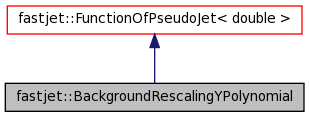FastJet 3.0.4
fastjet::BackgroundRescalingYPolynomial Class Reference

A background rescaling that is a simple polynomial in y. More...

#include <fastjet/tools/BackgroundEstimatorBase.hh>

Inheritance diagram for fastjet::BackgroundRescalingYPolynomial:[legend]
Collaboration diagram for fastjet::BackgroundRescalingYPolynomial:[legend]

List of all members.

Public Member Functions

BackgroundRescalingYPolynomial (double a0=1, double a1=0, double a2=0, double a3=0, double a4=0)
construct a background rescaling polynomial of the form a0 + a1*y + a2*y^2 + a3*y^3 + a4*y^4
virtual double result (const PseudoJet &jet) const
return the rescaling factor associated with this jet

Detailed Description

A background rescaling that is a simple polynomial in y.

Definition at line 181 of file BackgroundEstimatorBase.hh.

Constructor & Destructor Documentation

 fastjet::BackgroundRescalingYPolynomial::BackgroundRescalingYPolynomial ( double a0 = 1, double a1 = 0, double a2 = 0, double a3 = 0, double a4 = 0 ) [inline]

construct a background rescaling polynomial of the form a0 + a1*y + a2*y^2 + a3*y^3 + a4*y^4

The following values give a reasonable reproduction of the Pythia8 tune 4C background shape for pp collisions at sqrt(s)=7TeV:

• a0 = 1.157
• a1 = 0
• a2 = -0.0266
• a3 = 0
• a4 = 0.000048

Definition at line 196 of file BackgroundEstimatorBase.hh.

The documentation for this class was generated from the following files: